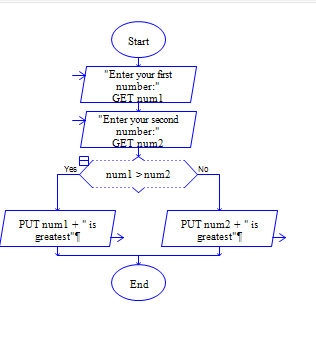# Greatest of two numbers in Python

Python Program to get two numbers num1 and num2 and find the greatest one among num1 and num2.

Sample Input 1:

5 6

Sample Output 1:

6

#### Flow Chart DesignStrongly recommended to Solve it on your own, Don't directly go to the solution given below.

#### Program or Solution

``` num1=int(input("Enter your first number:")) num2=int(input("Enter your second number: ")) if(num1>num2): print("{} is greatest".format(num1)) elif(num2>num1): print("{} is greatest".format(num2)) else: print("{} and {} are equal".format(num1,num2)) ```

#### Program Explanation

Get two inputs num1 and num2 from user using input() method check whether num1 is greater than num2 using if statement.

if num1 is greater print num1 using print() method, else check whether num2 is greater than num1 using elseif statement.

If num2 is greater print num2 using print() method, else print num1 and num2 are equal using print() method.